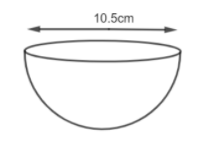Courses
Courses for Kids
Free study material
Free LIVE classes
MoreLIVE
Join Vedantu’s FREE Mastercalss

# A hemispherical bowl made of brass has an inner diameter of 10.5 cm. Find the cost of tin – plating it on the inside at the rate of Rs. 16 per 100 $c{{m}^{2}}.$.Verified
333.9k+ views
Hint: We have the diameter of the hemispherical bowl as 10.5 cm. We can use the formula $r=\dfrac{D}{2}$ to find the radius. Then using the radius, we can find the surface area of the hemisphere using $S=2\pi {{r}^{2}}.$ Once we have the surface area, the total cost is given by multiplying it with the cost per $c{{m}^{2}}.$

We are given a hemisphere whose inner diameter is 10.5 cm.We will first find the radius. We know that diameter is defined as two times the radius.
$D=2r$
So dividing both the sides by 2, we get,
$\Rightarrow r=\dfrac{D}{2}$
We are given that diameter = 10.5. So,
$D=10.5cm$
$\Rightarrow r=\dfrac{D}{2}$
$\Rightarrow r=\dfrac{10.5}{2}$
$\Rightarrow r=5.25cm$
Now, to find the cost, first, we will find the inner curved surface area of the hemisphere. We know that the surface area of the hemisphere is given as
$S=2\pi {{r}^{2}}$
Here, r = 5.25 cm. So, we get,
$\Rightarrow S=2\times \pi \times {{\left( 5.25 \right)}^{2}}$
Expanding the above terms, we get,
$\Rightarrow S=2\times \dfrac{22}{7}\times 5.25\times 5.25$
Solving the above equation further, we get,
$\Rightarrow S=173.25c{{m}^{2}}$
Therefore, the surface area of tin plating $=173.25c{{m}^{2}}.$
Now, we are given that the cost of tin plating is Rs. 16 for $100c{{m}^{2}}.$ That is,
$Rs.16=100c{{m}^{2}}$
So by the unitary method, we will find the cost of $1c{{m}^{2}}.$
As
$100c{{m}^{2}}=Rs.16$
$\Rightarrow 1c{{m}^{2}}=Rs.\dfrac{16}{100}$
Now, we will find for $173.25c{{m}^{2}}.$
$\Rightarrow 173.25c{{m}^{2}}=\dfrac{16}{100}\times 173.25$
$=Rs.27.72$
Therefore, the cost of tin plating the inner hemispherical bowl is Rs. 27.72.

Note: Students should not forget to convert the diameter to the radius, then only we could find the surface area. Also, do not multiply the surface area with 16, which is the total cost for 100. So, we have to divide it with 100 as well, then only we will get our required answer, else we would end up with the wrong answer.

Last updated date: 20th Sep 2023
Total views: 333.9k
Views today: 4.33k